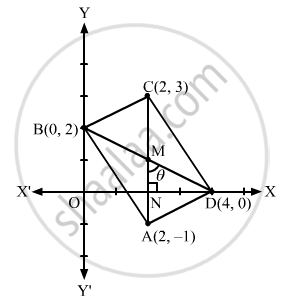Department of Pre-University Education, KarnatakaPUC Karnataka Science Class 11
Advertisement Remove all ads

# Prove that the Points (2, −1), (0, 2), (2, 3) and (4, 0) Are the Coordinates of the Vertices of a Parallelogram and Find the Angle Between Its Diagonals. - Mathematics

Answer in Brief

Prove that the points (2, −1), (0, 2), (2, 3) and (4, 0) are the coordinates of the vertices of a parallelogram and find the angle between its diagonals.

Advertisement Remove all ads

#### Solution

Let A(2, −1), B(0, 2), C(2, 3) and D(4, 0) be the vertices.
Slope of AB = $\frac{2 + 1}{0 - 2} = - \frac{3}{2}$

Slope of BC = $\frac{3 - 2}{2 - 0} = \frac{1}{2}$

Slope of CD = $\frac{0 - 3}{4 - 2} = - \frac{3}{2}$

Slope of DA = $\frac{- 1 - 0}{2 - 4} = \frac{1}{2}$

Thus, AB is parallel to CD and BC is parallel to DA.
Therefore, the given points are the vertices of a parallelogram.Now, let us find the angle between the diagonals AC and BD.
Let $m_1 \text { and } m_2$ be the slopes of AC and BD, respectively.

$\therefore m_1 = \frac{3 + 1}{2 - 2} = \infty$

$m_2 = \frac{0 - 2}{4 - 0} = - \frac{1}{2}$

Thus, the diagonal AC is parallel to the y-axis.

$\therefore \angle ODB = \tan^{- 1} \left( \frac{1}{2} \right)$

In triangle MND,

$\angle DMN = \frac{\pi}{2} - \tan^{- 1} \left( \frac{1}{2} \right)$

Hence, the acute angle between the diagonal is $\frac{\pi}{2} - \tan^{- 1} \left( \frac{1}{2} \right)$.

Is there an error in this question or solution?
Advertisement Remove all ads

#### APPEARS IN

RD Sharma Class 11 Mathematics Textbook
Chapter 23 The straight lines
Exercise 23.13 | Q 3 | Page 99
Advertisement Remove all ads

#### Video TutorialsVIEW ALL 

Advertisement Remove all ads
Share
Notifications

View all notifications

Forgot password?